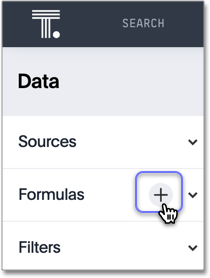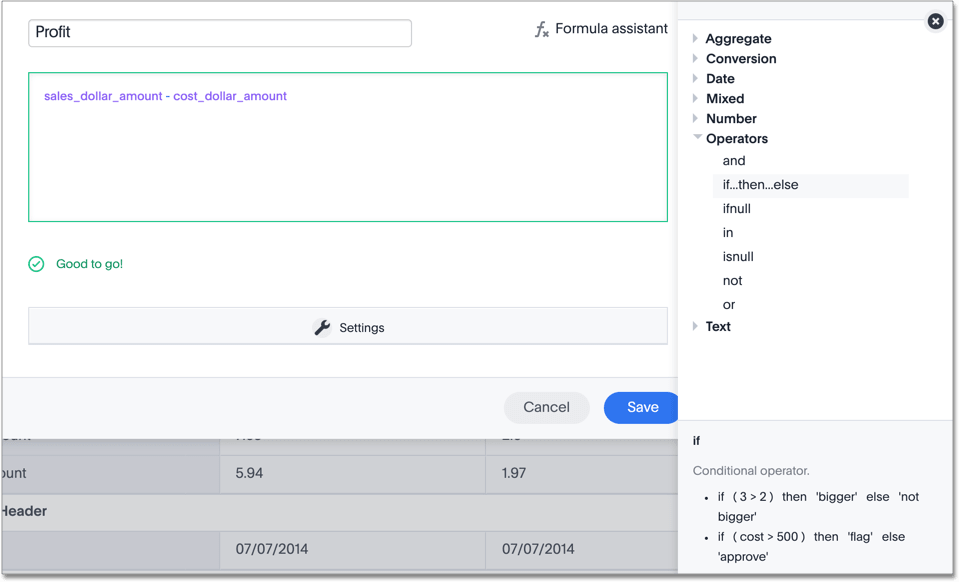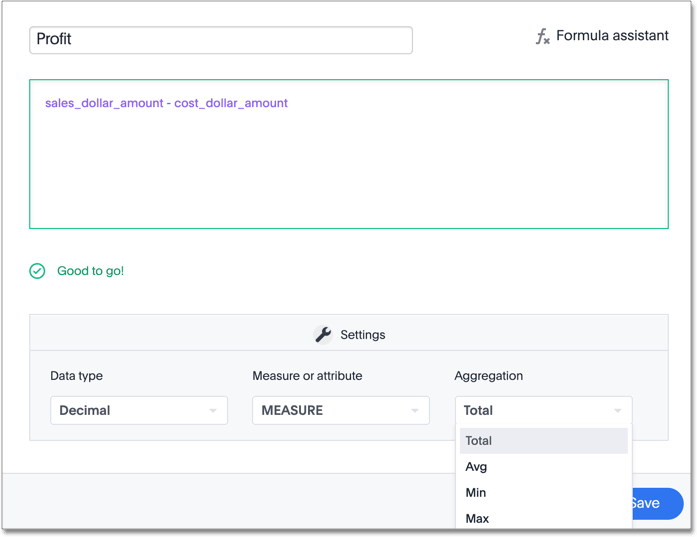# Create a formula in a Worksheet

You create formulas by combining standard functions and operators, column names, and constant values.

Anyone who can create a Worksheet can add a formula to it. Formulas are not reusable; the formula you create is associated only with the Worksheet it belongs to. A complete list of available formulas and examples of each is available in the Formula function reference.

You can create a formula in a Worksheet by using the Formula Builder. When you do this, the result of the formula gets added to the Worksheet as a column. Use these steps to create a formula:

1. Select the + button next to Formulas.2. Type your formula in the Formula Builder.Formula elements are color coded by type and can include the formula operators and functions (blue), the names of columns (purple), and/or constants (black).
3. You can see a list of formula operators with examples by selecting on Formula Assistant.4. If you want to change what your formula returns, use the Advanced settings.

Depending on your formula, you may be able to change:

• Data type: Determines the type of data that the formula generates. For example, use `date` for an `add_days` formula.

• Measure or attribute: Determines if the output of the formula is a measure or an attribute. For example, choose `attribute` for a formula that generates age groups, and choose `measure` for a formula that generates profit.

• Aggregation: Determines the default aggregation of the formula output. For example, choose `min` to see minimum profit.5. Name the formula by selecting on its title and typing the new name. Select Save.